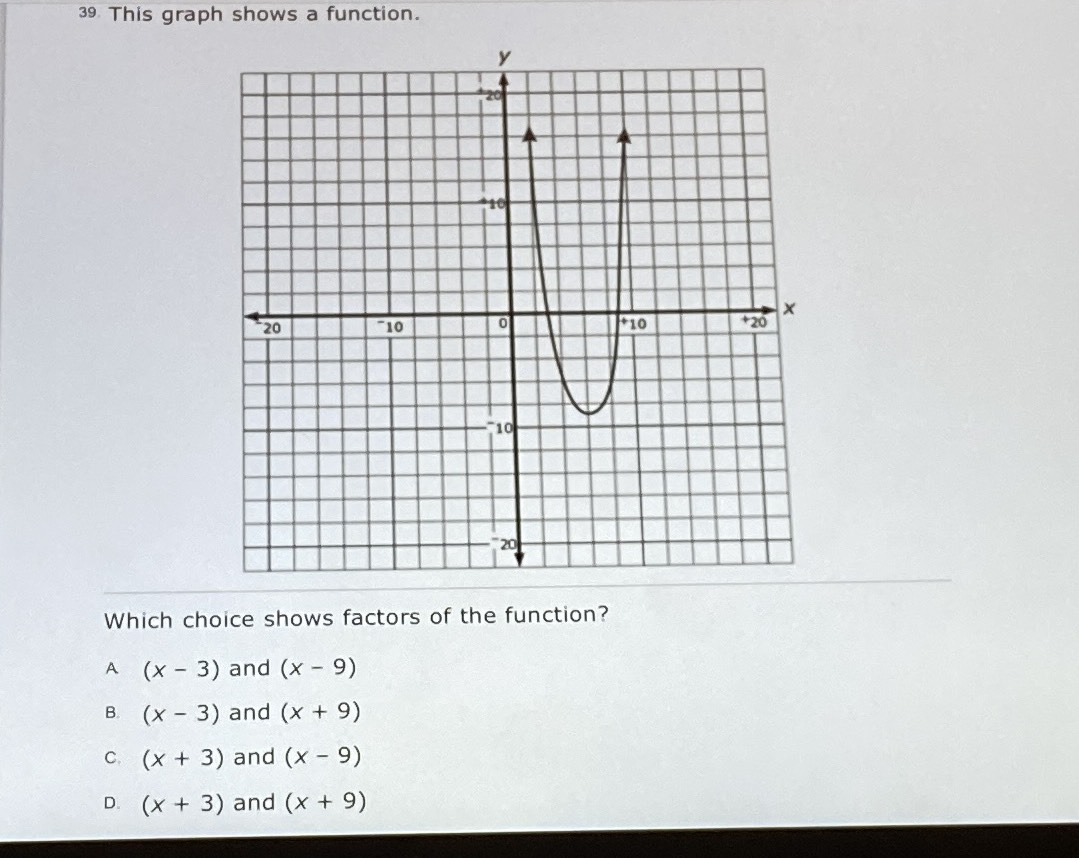### ¿Todavía tienes preguntas de matemáticas?

Pregunte a nuestros tutores expertos
Algebra
Pregunta39. This graph shows a function. Which choice shows factors of the function?

$$( x - 3 )$$ and $$( x - 9 )$$

B. $$( x - 3 )$$ and $$( x + 9 )$$

C. $$( x + 3 )$$ and $$( x - 9 )$$

D. $$( x + 3 )$$ and $$( x + 9 )$$